# Recent Research Papers

## Robert L. Devaney

### Illuminating the Mandelbrot Set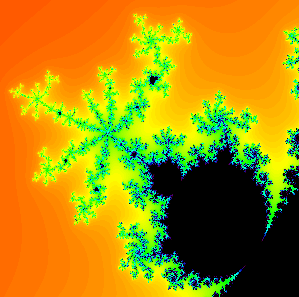##### The 3/7-bulb hanging off the main cardioid in the Mandelbrot set. You can read off "3/7" by just looking at how the spokes are arranged in the antenna attached to this bulb. Or maybe you can just read this paper...
This paper is a summary of a lecture I gave on the fractal geometry of the Mandelbrot set at a conference at Mar-Athanasius College in India. To appear in the Proceedings of the Seminar on Topology and Fractals from that conference.

### Mandelpinski Necklaces in the Parameter Planes of Rational Maps

With Sebastian M. Marotta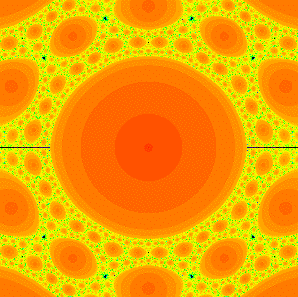##### Mandelpinski necklaces surrounding the McMullen domain in the family z3 + C/z3. The red disks are Sierpinksi holes, and the black "dots" are really baby Mandelbrot sets.
This paper is a survey of some recent results involving Mandelpinski Necklaces for the family of rational maps zn + C /zd. The main emphasis is on the case where n = d > 2, but the other cases are treated briefly. In Topological Dynamics and Topological Data Analysis, Springer (2021), 95-119.

### Discrete Dynamical Systems: A Pathway for Students to Become Enchanted with Mathematics##### The Sierpinski carpet, generated by playing the chaos game with eight vertices arranged at the ends and centers of the four sides of a square, with compression ratio 3 and no rotations.
This paper is a pedagogical article aimed at showing high school teachers how topics from dynamical systems can be incorporated into the curriculum in order to get students excited about mathematics. Most of the paper centers around the chaos game and its variants. In Teaching and Learning Discrete Mathematics Worldwide: Curriculum and Research, Springer ICME-13 Monographs (2018), 137-144.

### Mandelpinski Structures in the Parameter Planes of Rational Maps##### A different zoom into the Mandelpinski maze structure for the family of rational maps z2 + C / z3. The Mandelbrot sets are too small to be seen, but the red disks are Sierpinski holes.
In this paper we give a survey of several different "Mandelpinski structures" that arise in the families of rational maps zn + C / zd where n, d > 1. These structures include Mandelpinski necklaces, Mandelpinski spokes, and a Mandelpinski maze. Ful details are provided in the papers below. In Proceedings of the Oxtoby Centennial Conference, AMS Contemporary Math Series 678 (2016), 133-150.

### Mandelpinski Spokes in the Parameter Planes for Rational Maps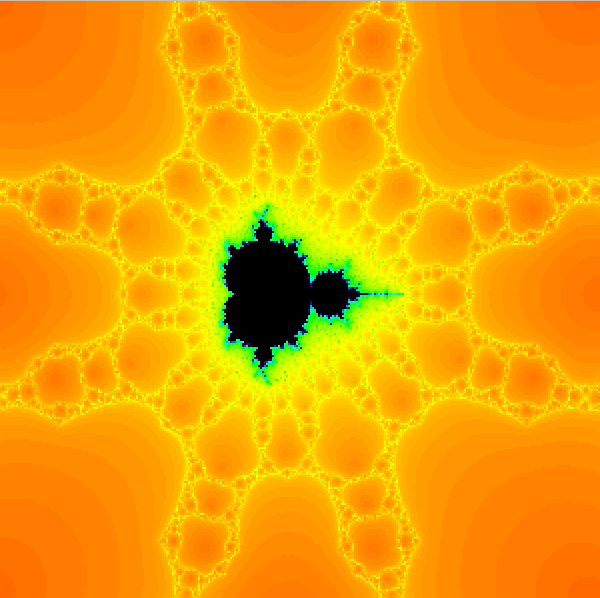##### Some Mandelpinski spokes emanating from a baby Mandelbrot set in the parameter family of z2 + C / z3. The Mandelbrot sets are too small to be seen, but the red disks are Sierpinski holes.
In this paper we describe another type of structure in the parameter plane for rational maps of the form zn + C / z d where n > 1 is even and d > 2 is odd. These are Mandelpinski spokes. Each spoke is an infinite collection of alternating Mandelbrot sets and Sierpinski holes that extend away from certain Mandelbrot sets in the Mandelpinski maze described in the following paper. Journal of Difference Equations and Applications 22 (2016), 330-342.

### A Mandelpinski Maze for Rational Maps of the Form zn + C/zd.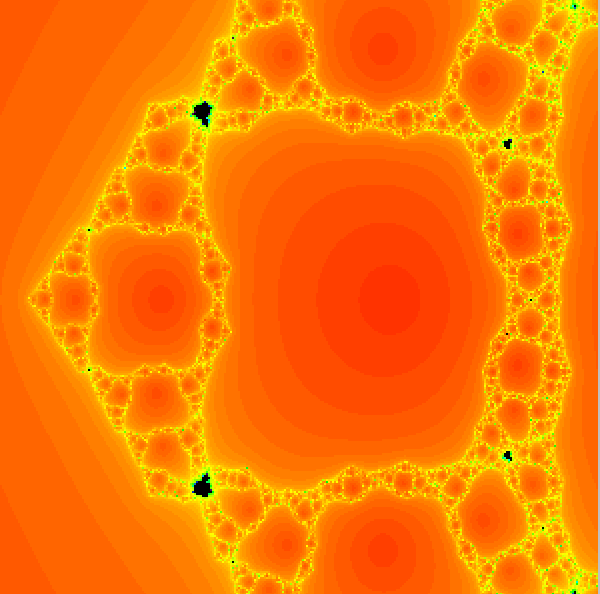##### A portion of the Mandelpinski maze along the negative real axis for z2 + C / z3. The Mandelbrot sets are too small to be seen, but the red disks are Sierpinski holes.
In this paper we describe a new type of structure in the parameter plane for rational maps of the form zn + C / z d where n > 1 is even and d > 2 is odd that we call a Mandelpinski maze. The maze is an infinite collection of alternating Mandelbrot sets and Sierpinski holes arranged along a complicated planar graph with infinitely many vertices. Indagationes Mathematicae 27 (2016), 1042-1058.

### Exotic Topology in Complex Dynamics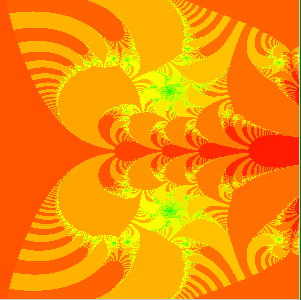##### The Julia set for the complex exponential family C exp(z) when C > 1/e contains indecomposable continua.
This paper is a brief overview of some of the interesting topological spaces that arise as Julia sets for complex analytic maps. Included are Cantor bouquets, indecomposable continua, and Sierpinski curves. This is basically an outline of a lecture given at a workshop on fractals at the Rajagiri School of Engineering and Technology in Kochi, India in 2013 and at the conference on Geometry and Dynamics at the Lorentz Center in Leiden, Netherlands in 2015. Indagationes Mathematicae 27 (2016), 1116-1126.

### Accessible Mandelbrot Sets in the Family zn + C/zn

With Paul Blanchard, Daniel Cuzzocreo, and Elizabeth Fitzgibbon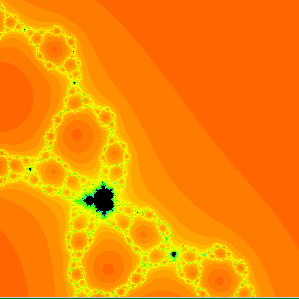##### A magnification of the parameter plane for the family of maps z4 + C / z4 showing some accessible baby Mandelbrot sets.
In this paper we prove that there exist infinitely many accessible baby Mandelbrot set in the parameter plane for the family of rational maps zn + C / zn where n > 1. These are Mandelbrot sets whose main cusp touches the exterior boundary of the connectedness locus in the parameter plane. We also show that there is one such Mandelbrot set for each external ray of angle j / nk - 1. Qualitative Theory of Dynamical Systems 15 (2016), 49-66.

### A Dynamical Invariant for Sierpinski Cardioid Julia Sets

With Paul Blanchard, Daniel Cuzzocreo, Elizabeth Fitzgibbon, and Stefano Silvestri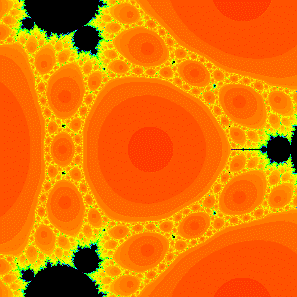##### A magnification of the parameter plane for the family of maps z4 + C / z4 showing the Mandelpinski necklaces surrounding the central region which is the McMullen domain.
In this paper we give a dynamical invariant for the Sierpinski curve Julia sets that lie in the main cardioids of the baby Mandlebrot sets attached to the Mandelpinski necklaces surrounding the McMullen domain for the family zn + C / zn when n > 2. This invariant is basically the itinerary of the periodic critical orbit as it winds through the various sectors bounded by Cantor necklaces. Fundamenta Mathematicae 226 (2014), 253-277.

### A Cantor-Mandelbrot-Sierpinski Tree in the Parameter Plane for Rational Maps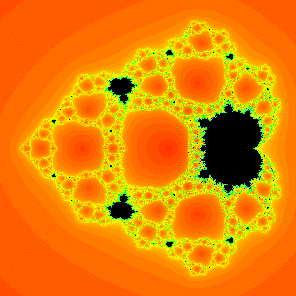##### The parameter plane for the family of functions z2 + C / z2 showing the CMS tree with the main trunk along the horizontal axis.
In this paper we construct what we call a Cantor-Mandelbrot-Sierpinski tree in the parameter plane for the family of rational maps z2 + c/z2. This is a "tree" whose trunk and infinitely many branches are all Cantor necklaces. So most of the parameters in these regions have Julia sets that are Sierpinski curves. Meanwhile, between each pair of branches there is also a baby Mandelbrot set extending outward from the main trunk of the tree. Transactions of the AMS 366 (2014), 1095-1117.

### Sierpinski Curve Julia Sets for Quadratic Rational Maps

With Núria Fagella, Antonio Garijo, and Xavier Jarque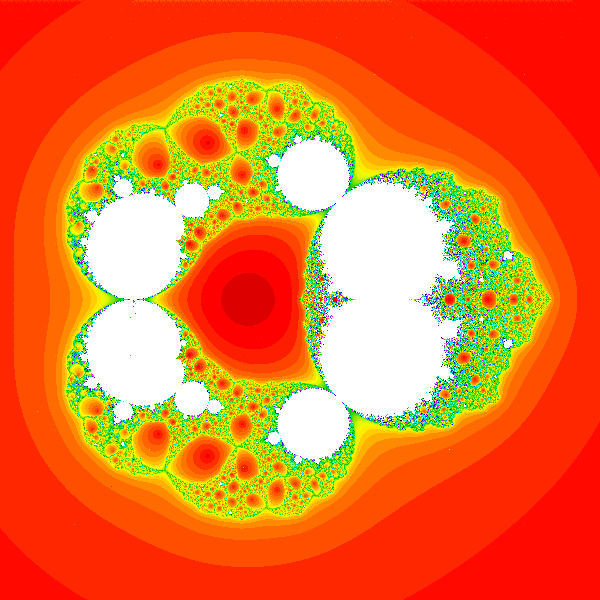##### A slice of the parameter space for rational maps of degree 2.
In this paper we give an almost complete set of conditions for a hyperbolic rational map of degree two to have a Julia set that is a Sierpinski curve. Annales Acadademiae Scientarum Fennicae 39 (2014), 3-22.

### Julia Sets Converging to Filled Quadratic Julia Sets

With Robert Thijs Kozma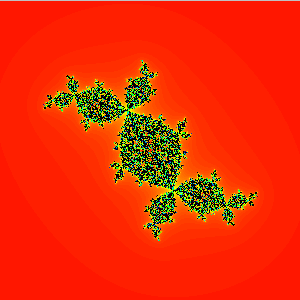##### The Julia set corresponding to a singular perturbation of the Douady rabbit.
In this paper we consider maps of the form FA(z) = z2 + c + A / z2 where c is the center of a hyperbolic component in the Mandelbrot set. We prove that, as A tends to 0, the Julia set of FA tends to the FILLED Julia set of F0, i.e., the filled Julia set of the simple quadratic function z2 + c. This generalizes the result with Toni Garijo (below) where this was shown for c = 0 and also the result with Mark Morabito (below) where maps of the form zn + A/z were shown to have similar behavior, at leasst when A approached the origin along n-1 straight lines. Finally, in the paper A Generalized Version of the McMullen Domain (also below), it was shown that this does not happen in the family FA(z) = zn + c + A / zn where c is the center of a hyperbolic component of the Multibrot set and n > 2. Ergodic Theory and Dynamical Systems 34 (2014), 171-184.

### Singular Perturbations of Complex Polynomials##### The McMullen domain for z3 + C / z3. This disk is surrounded by infinitely many Mandelpinski necklaces.
This paper is a survey of much of the work that I have been doing over the past few years involving the family of rational maps zn + A/zd. Included are overviews dealing with the escape trichotomy, Sierpinski curve Julia sets, Mandelpinski necklaces, Cantor webs and necklaces, the crazy case n = d = 2, more general singularly perturbed maps, and a host of open problems. Bulletin of the American Math. Society 50 (2013), 391-429.

### A Century of Complex Dynamics

With Dan Alexander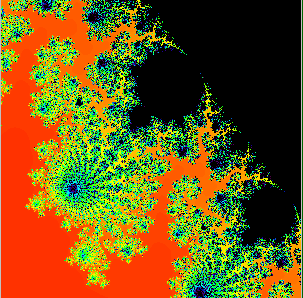##### A period 43 bulb off the main cardioid. How do you know this? Just count the spokes of the antenna hanging off the bulb. Can't count that high? See the paper below.
This paper is a brief history of the past one hundred years in the field of complex dynamics. It also gives a brief introduction to such objects as Julia sets and the Mandelbrot set. It is written to coincide with the centennial of the Mathematical Association of America, which occurs in 2015. In A Century of Advancing Mathematics, MAA (2015), 15-34.

### Parameter Planes for Complex Analytic Maps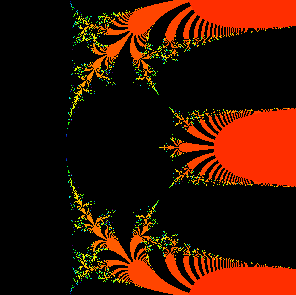##### The main cardioid in the parameter plane for the family C exp(z).
This paper is a brief overview of some structures in the parameter planes for complex quadratic polynomials (the Mandelbrot set), singularly perturbed rational maps, and the complex exponential. It is basically an outline of a series of lectures given at a workshop on fractals at the Rajagiri School of Engineering and Technology in Kochi, India in 2013. In Fractals, Wavelets, and their Applications. Springer-Verlag (2014) 61-77.

### The Complex Geometry of the Mandelbrot Set

v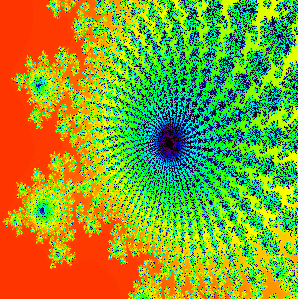##### A magnification of the antennas in a period 43 bulb hanging off the main cardioid. How do you know this? Just count the spokes of the antenna hanging off the bulb. Maybe this is easier now than above.
This paper is a brief survey of the structure of the principal bulbs hanging off the main cardioid of the Mandelbrot set. Each of these bulbs has its own "identity," and, as long as you know how to add and how to count, you can identify each such bulb. Now I know many of you think you know how to add, but this paper will convince you that you are wrong! This is a summary of a lecture I gave at the Complex Systems Conference in Prague in 2013. and that will appear in the Proceedings of that conference. In ISCS 2013: International Symposium on Complex Systems. Springer-Verlag (2014) 3-8.

### My Favorite Planar Fractal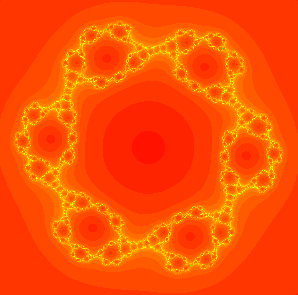##### A Sierpinski carpet Julia set coresponding to the map z3 + (-.2 + 11i) / z3.
This paper is just a brief, one-page note giving three reasons why my favorite planar fractal is the Sierpinski carpet, not the triangle (or gasket). Canadian Math Society Notes. 45 No. 3 (2013), 13.

### Simple Mandelpinski Necklaces for z2 + C / z2.

With Daniel Cuzzocreo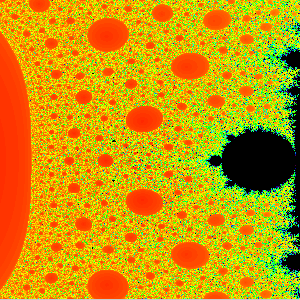##### A magnification of the parameter plane for the family of maps z2 + C / z2. The Mandelpinski necklaces here are not as evident as they are in the case where n > 2.
In this paper we return to the construction of Mandelpinski necklaces that were proved to exist for the family of rational maps zn + C / zn when n > 2 in several papers below. Here we extend these results to the very different case n = 2. One main difference is that there is no McMullen domain in this case, so the necklaces converge to the origin in the parameter plane. Also, each of these special necklaces only pass through one Mandelbrot set and one Sierpinski hole. When n > 2, these necklaces pass through many more such sets. In Difference Equations, Discrete Dynamical Systems and Applications. Springer-Verlag (2016), 63-72.

### Connectivity of Julia Sets for Singularly Perturbed Rational Maps

With Elizabeth D. Russell##### The connected Julia set for the map z3 + (.105 + .05i) / z2. Note the Douady rabbits that make up a portion of the Julia set for this map.
This paper augments the results in the paper The Escape Trichotomy for Singularly Perturbed Rational Maps (below). We show here that, for the family zn + C / zn, the Julia set is always a connected set, except in two cases: the case where the Julia set is a Cantor set and the case where it is a Cantor set of simple closed curves. In Chaos, CNN, Memristors and Beyond, World Scientific (2013), 239-245.

### Limiting Behavior of Julia Sets of Singularly Perturbed Rational Maps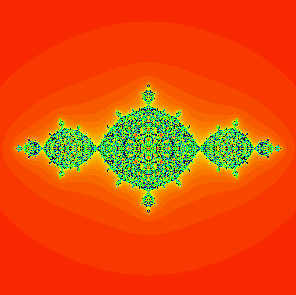##### The Julia set for the map z2 -1 + A / z2. These Julia sets converge to the filled Julia set known as the basilica as A approaches 0.
This paper is a survey of the different things that happen to the Julia sets in the families zn + c + A/zd as the parameter A approaches 0 and c is at the center of a hyperbolic component of the Multibrot set. When n = d = 2, we describe how the Julia sets limit on the filled Julia set of z2 + c. Then we show that this does not occur for most other cases. In Frontiers of Complex Dynamics: In Celebration of John Milnor's 80th Birthday. Princeton University Press (2014), 121-134.

### Checkerboard Julia Sets for Rational Maps

With Paul Blanchard, Figen Cilingir, Daniel Cuzzocreo, Daniel M. Look, and Elizabeth D. Russell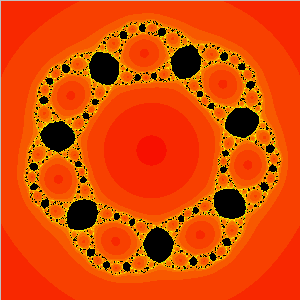##### A checkerboard Julia set corresponding to a map of the form z4 + C / z3.
In this paper we describe the dynamics of maps of the form maps of the form zn + C / zd where n > 1 and d > 0 and C is drawn from the main cardioid of one of the principal Mandelbrot sets in the parameter plane. These Julia sets (called checkerboard Julia sets) are all homeomorphic but not all dynamically equivalent. We prove that two such maps have conjugate dynamics if and only if they have the same "minimal rotation number." In Int'l J. Bifurcation & Chaos 23 (2013) 48-60.

### Dynamics of zn + C/zn; Why the Case n = 2 is Crazy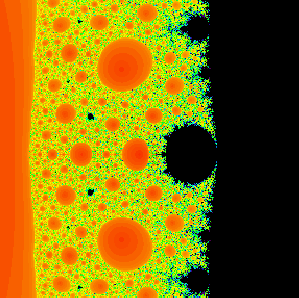##### The parameter plane for the family of maps z2 + C / z2. There is no McMullen domain around 0 when n = 2.
In this paper we give three examples of how the dynamics of maps of the form zn + C / zn are most complicated when n = 2. There are three reasons for this. First, there is no McMullen domain in this case, and secondly, because of this, there are no Mandelpinski necklaces surrounding the origin. Third, as C goes to 0, the Julia sets converge to the closed unit disk when n = 2, but this does not occur for n > 2. In Conformal Dynamics and Hyperbolic Geometry, Contemporary Math. AMS Vol. 573 (2012), 49-65.

### Extending External Rays Throughout the Julia Sets of Rational Maps

With Figen Cilingir and Elizabeth D. Russell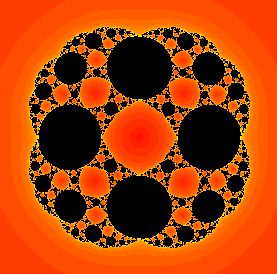##### The Julia set for the map z2 + .08 / z2. The extended rays for this map are all simple closed curves passing through 0 and infinity that meet the Julia set in a Cantor set.
In this paper we give three examples of how external rays in the dynamical plane may be extended completely through the Julia sets of certain rational maps. These are maps of the form zn + C / zn. In this case most of these extended rays are simple closed curves passing through the origin (a pole) and infinity. They also cut across the Julia set in a Cantor set of points. In certain cases, these extended rays meet a critical orbit, in which case they bifurcate into simple closed curves with (possibly infinitely many) attached arcs. In Smale Festschrift, J. Fixed Point Theory and Applications 7 2010, 223-240.

### Intertwined Internal Rays in Julia Sets of Rational Maps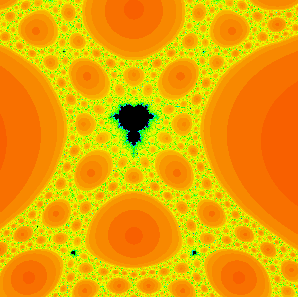##### A baby Mandelbrot set in the family of degree 6 maps z3 + C / z3.
In this paper we extend the concept of a dynamical plane external ray to that of an internal ray. For a Cantor set of angles on the unit circle, this is a curve that threads its way through the Julia set of a map of the form zn + C/zn where n > 2. Unlike external rays, all these internal rays cross other internal rays at infinitely many points, and how these crossings are arranged determine to a large part the structure of the Julia set. In particular, these internal rays allow us to prove the existence of (n-2)(2n-2)k-1 baby Mandelbrot sets of base period k in the parameter plane for these families of rational maps. Fundamenta Mathematicae 206 2009, 139-159.

### Singular Perturbations of Complex Analytic Dynamical Systems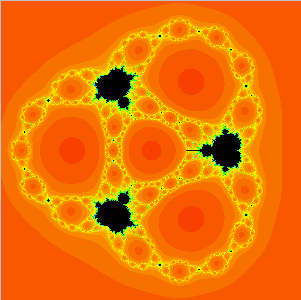##### The parameter plane for the family of degree 8 maps z4 + C / z4.
This paper is a survey of many of the results in the papers below dealing with singularly perturbed rational maps. We concentrate for simplicity on the family zn + c / zn where the parameter c is complex. We describe the Escape Trichotomy Theorem, the classification of escape time Sierpinski curve Julia sets, the structure around the McMullen domain, Cantor necklaces, and Julia sets converging to the unit disk. A subtheme in the paper is how the case n = 2 is very different from the case n > 2. In Nonlinear Dynamics and Chaos: Advances and Perspectives, Springer-Verlag, Berlin, 2010, 13-29.

### Dynamic Classification of Escape Time Sierpinski Curve Julia Sets

With Kevin Pilgrim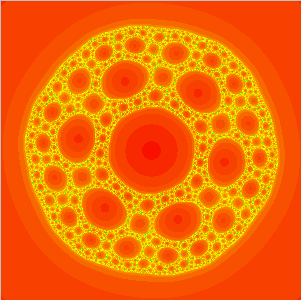##### The Julia set for z3 - i + (-.025 + .034i) / z3 that is the center of a Sierpinski hole. Only the map with a complex conjugate parameter is conjugate to this one.
In this paper, we consider families of maps of the form zn + c/zn where the parameter c is a center of a Sierpinski hole in the parameter plane. So each of these Julia sets is homeomorphic to the Sierpinski carpet and hence to each other. We give a complete dynamic classification of these "escape time Sierpinski curve maps," i.e., those maps for which the free critical orbits escape. In particular, we show that there are exactly (2n)k-3 different conjugacy classes when n is odd and (2n)k-3 + 2k-4 classes when n is even. Here k is the escape time of the critical orbits. It is known that there are exactly (n-1) (2n)k-3 such centers of Sierpinski holes, so very few of these maps have conjugate dynamics. The result holds for all maps in a Sierpinski hole, not just for the centers. Fundamenta Mathematicae 202 (2009), 181-198.

### A Generalized Version of the McMullen Domain

With Paul Blanchard, Antonio Garijo, and Elizabeth D. Russell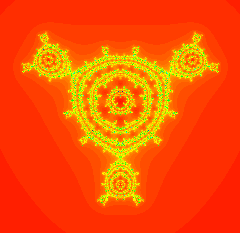##### The Julia set for z3 - i + A / z3 containing a Cantor set of decorated circles.
In this paper, we consider families of maps of the form zn + c +A/zn where the parameter A is a center of a hyperbolic component of the Multibrot set and n > 2. We show that, for A sufficiently small the Julia set of this map contains a countable collection of simple closed curves (some of which are "decorated") together with an uncountable collection of point components. This generalizes a result of McMullen which shows that, when A = 0, the Julia set is a single Cantor set of simple closed curves. International Journal of Bifurcation and Chaos 18 (2008), 2309-2318.

### Limiting Julia Sets for Singularly Perturbed Rational Maps

With Mark Morabito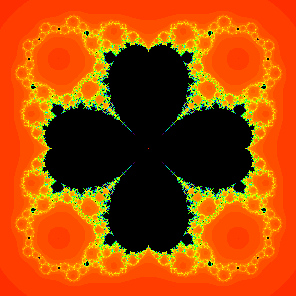##### The parameter plane in the case n=5, showing in color the four dividing rays tending to the origin on which the Julia sets converge to the unit disk.
In this paper, we consider families of maps of the form zn + A/z where the parameter A is drawn from n - 1 special dividing rays in the parameter plane. We show that, as A approaches the origin along these rays, the Julia sets converge as a set to the closed unit disk. This paper extends the results in the paper below entitled Julia Sets Converging to the Unit Disk. International Journal of Bifurcation and Chaos 18 (2008), 3175-3181.

### Rabbits, Basilicas, and Other Julia Sets Wrapped in Sierpinski Carpets

With Paul Blanchard, Antonio Garijo, Sebastian Marotta, and Elizabeth D. Russell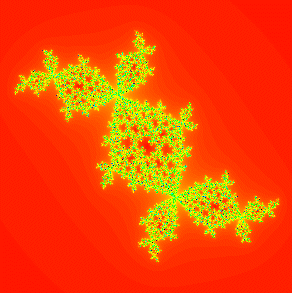##### A singular perturbation of the Douady rabbit. The interior of each component of the filled Julia set of the rabbit contains a Sierpinski curve.
In this paper, we consider families of maps of the form z2 + c + A/z2 where c is the center of a hyperbolic component of the Mandelbrot set with period greater than 1 and A is a small complex parameter. We show that, if |A| is sufficiently small, then the Julia set of these maps are bounded on the outside by a homeomorphic copy of the Julia set of z2 + c. Also, assuming all of the critical orbits of the map eventually enter the basin of infinity, then each internal component of filled Julia set of this map is "wrapped" in a set homeomorphic to the Sierpinski carpet. In Complex Dynamics: Families and Friends, A. K. Peters (2009), 277-296.

### Chaos, Fractals, and Tom Stoppard's Arcadia##### The Mandelbrot set, or, as it is known in Arcadia, the "Coverly" set.
Well, this is not really a research paper. Rather, it is a summary of some of the mathematical ideas that arise in the wonderful play by Tom Stoppard called Arcadia. Over the years, I have been the "chaos consultant" for several theaters' production of this play, and I find that people involved with this play often want to learn a bit more about the mathematical ideas involving chaos and fractals that swirl throughout the play. This paper is an attempt to introduce these ideas at a relatively low level and in a manner that is relevant to the various scenes in the play. In The Beauty of Fractals: 6 Different Views, Mathematical Association of America. Eds. Jon Scott and Dennis Gulick. (2010).

### Julia Sets Converging to the Unit Disk

With Antonio Garijo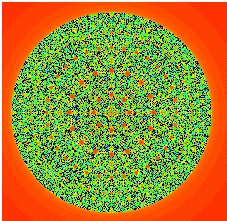##### The Julia set for F(z) = z2 -0.00001 / z2 is very close to the unit disk.
In this paper we study the behavior of the Julia sets of the maps Fc(z) = zn + c / zd where n and d are both greater than or equal to 2 and c is small. When n = d = 2, we prove that the Julia sets of these maps converge as c tends to 0 to the unit disk in the plane. But when one of n or d is greater than 2, we show that there is always an annulus of some fixed size that separates portions of the Julia set. So the Julia sets do not converge to the unit disk in this case. Proceedings of the American Mathematical Society 136 (2008), 981-988.

### Complex Exponential Dynamics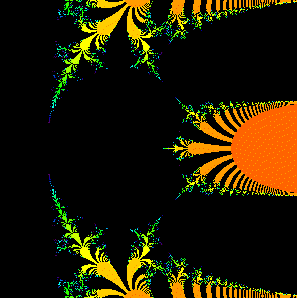##### A portion of the bifurcation diagram (the analogue of the Mandelbrot set) for the complex exponential function C ez
This paper is a survey of complex exponential dynamics. Topics covered include preliminaries on complex dynamics, Cantor bouquets, indecomposable continua, the parameter plane, structural instability, the relation between the exponential and quadratic (and other polynomial) dynamics, and some comparisons with other important families of entire and meromorphic maps. In Handbook of Dynamical Systems, Vol. 3. Eds. H. Broer, F. Takens, B. Hasselblatt. 2010, 125-224.

### Cantor Sets of Circles of Sierpinski Curve Julia Sets##### The McMullen domain for z3 + C / z3. The Cantor set of circles of Sierpinski curve Julia sets weaves its way in between the visible curves that contain collections of Sierpinski holes.
In this paper we prove that, in the parameter plane for the family of maps given by z3 + C / z3, there is a Cantor set of simple closed curves on which each parameter corresponds to a map whose Julia set is a Sierpinski curve. Hence all these Julia sets are homeomorphic. However, only finitely many of these maps are conjugate to another such map, so we have uncountably many dynamically different maps on these curves. Related results are also proved in the dynamical plane for the more general families zn + C / zn where n > 2. Ergodic Theory and Dynamical Systems 27 (2007), 1525-1539.

### Evolution of the McMullen Domain for Singularly Perturbed Rational Maps

With Sebastian M. Marotta##### The McMullen domain when n=2, d=3. Actually, the domain is invisible in this picture since it is so small. It lies at the tip of the tail of the Mandelbrot set at the right.
In this paper we consider families of maps of the form zn + C / (z - a)d where a and C are complex and |a| is not equal to one (and 1/n + 1/d < 1). We are especially interested in the case where a is close to 0. For when a = 0, there is a McMullen domain in the parameter space, but as soon as a becomes nonzero, this domain moves away from the origin and the Julia sets become very different. In the McMullen domain, the Julia sets are Cantor sets of concentric circles about the origin; when a is nonzero, the Julia set consists of infinitely many circles, only one of which surrounds the others, and uncountably many point components. Topology Proceedings 32 (2008), 301-320.

### Cantor Webs in the Parameter and Dynamical Planes of Rational Maps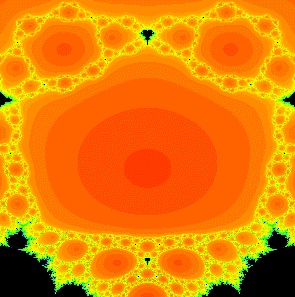##### A portion of the parameter plane for z3 + c / z3 showing a Cantor 4-web. The web is the union of many of the open regions plus a Cantor set of points.
In this paper we prove that there is a "Cantor 2n-2-web" in both the dynamical and the parameter plane for complex rational maps of the form zn + C / zn. Roughly speaking, a Cantor k-web consists of an open disk which is surrounded by k smaller open disks. Then each of these disks are surrounded by k smaller open disks. And so forth. Finally, this sturcture is joined together by adding a Cantor set of points that makes the entire set connected. In the dynamical plane, the dynamics of the map on each of these sets is always the same: on the Cantor set portion of the web, the map is conjugate to the shift map, while points in the open disks eventually escape to infinity. This structure occurs for all parameters c for which the Julia set is connected. Fields Institute Communications 53 (2008), 105-123.

### The McMullen Domain: Satellite Mandelbrot Sets and Sierpinski Holes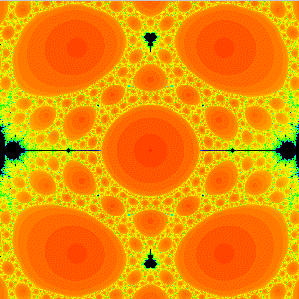##### A magnification of the McMullen domain in the parameter space for the family of rational maps z3 + A / z3. Note the "circles" meeting 4, then 10, then 28 Sierpinski holes surrounding the central McMullen domain.
In this paper we prove that the kth ring around the McMullen domain (as described in the paper below) for the family of rational maps zn + C / zn when n > 2 is the home of precisely (n - 2)nk-1 + 1 parameters that are centers of baby Mandelbrot sets of base period k. and the same number of parameter values that are centers of Sierpinski holes with escape time k+2. We also show that these Sierpinski holes are always homeomorphic to disks and that there is a unique parameter in each hole for which the critical orbit lands on infinity at iteration k+2. Finally, we show that there are exactly (2n)k-3(n-1) Sierpinski holes in the parameter plane with escape time k. These last two results are straightforward generalizations of results of Pascale Roesch, who proved these facts in the case n = 2. Conformal Geometry and Dynamics 11 (2007), 164-190.

### The McMullen Domain: Rings Around the Boundary

With Sebastian Marotta##### A magnification of the McMullen domain in the parameter space for the family of rational maps z4 + A / z4.
In this paper we prove that the McMullen domain for the family of rational maps zn + C / zn when n > 2 is surrounded by infinitely many disjoint simple closed curves Ck converging down to the boundary of the domain as k tends to infinity. On Ck, there are precisely
(n - 2)nk-1 + 1

parameters that are superstable (i.e., for which a critical orbit is periodic), and the same number of parameter values for which the critical orbits land on the pole at 0 (i.e., parameters that are centers of Sierpinski holes). Transactions of the American Mathematical Society 359 (2007), 3251-3273.

### Open Problems in Complex Dynamics and "Complex" Topology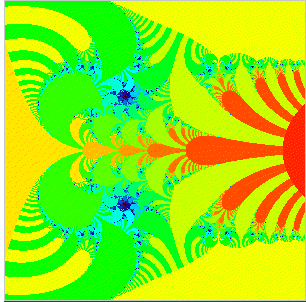##### The explosion that produces indecomposable continua in the family C exp(z) when C increases through 1 / e.
In this paper we give a survey of some open problems that occur on the "boundary" of complex dynamics and topology. Included are problems dealing with indecomposable continua and Sierpinski curve Julia sets that arise in complex dynamical systems. In Open Problems in Topology II, ed. Elliott Pearl, Elsevier (2007), 469-478.

### Singular Perturbations of zn

With Matt Holzer, Daniel M. Look, Mónica Moreno Rocha, and David Uminsky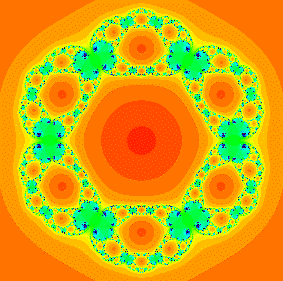##### A Julia set for a singularly perturbed function of the form z3 + C / z3.
In this paper we give a survey (with some proofs in elementary cases) of the vast array of different phenomena that occur in the family of rational maps
Fc(z) = zn + c / zd,
especially in the case where the parameter c is small. In Transcendental Dynamics and Complex Analysis, London Math Society Lecture Notes Series, Vol. 348, Cambridge University Press, eds. P. Rippon and G. Stallard, (2008) 111-137.

### Cantor Necklaces and Structurally Unstable Sierpinski Curve Julia Sets for Rational Maps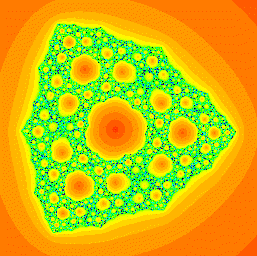##### An example of a structurally unstable Sierpinski curve Julia set from the family of functions z2 + C / z.
In this paper we show that the family of maps FA(z) = zn + A z-n with n > 1 admits infinitely many parameter values A for which the Julia set is a Sierpinski curve that is structurally unstable in the sense that, arbitrarily close to A, there are infinitely many parameter values for which:
• the Julia sets are structurally stable Sierpinski curves, but none are conjugate to each other or to FA;
• the Julia sets are structurally unstable Sierpinski curves, but none are conjugate to each other or to FA;
• the Julia sets are "hybrid" Sierpinski curves, but again, none are conjugate to each other or to FA.
Qualitative Theory of Dynamical Systems 5 (2006), 337-359.

### A Myriad of Sierpinski Curve Julia Sets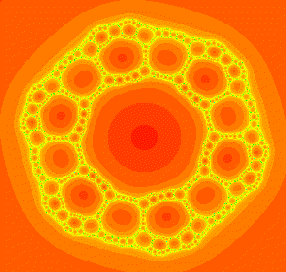##### A Sierpinski curve Julia set for the function z3 + 0.13i / z3.
In this paper we survey the many different ways that Sierpinski curves can appear as the Julia set of a member of the family of rational maps of the form zn + C / zd . In Difference Equations, Special Functions, and Orthogonal Polynomials. World Scientific (2007), 131-148.

### Rational Maps with Generalized Sierpinski Gasket Julia Sets

With Mónica Moreno Rocha and Stefan Siegmund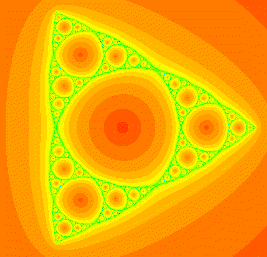##### The Julia set for the function z2 -0.592 / z is homeomorphic to the well-known Sierpinski gasket fractal.
In this paper we construct models for certain Julia sets in the families of rational maps z2 + A / z2 and z2 + B / z. These Julia sets are generalized Sierpinski gaskets and are produced when the critical orbits for these maps land on repelling periodic points in the boundary of the basin of infinity. We also show that, excluding a certain symmetry, no two of these Julia sets are homeomorphic. Topology and its Applications 154 (2007), 11-27.

### Unveiling the Mandelbrot Set##### The Mandelbrot set.
This paper is an elementary description of the Mandelbrot and Julia sets written for students who are familiar with complex numbers. Online in Plus Magazine, 2006.

### Blowup Points and Baby Mandelbrot Sets for Singularly Perturbed Rational Maps

With Matt Holzer and David Uminsky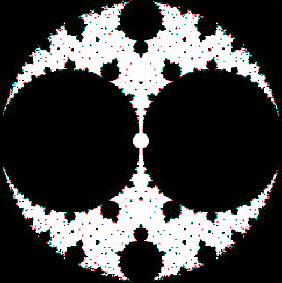##### The parameter plane for the family C(z + 1 / z).
In this paper we describe the structure of the parameter plane for the family of rational maps given by C(z + 1 / z). We show that in any neighborhood of C=0 there are infinitely many parameters for which the Julia set is the entire plane (blowup parameters) as well as infinitely many copies of small Mandelbrot sets. In Complex Dynamics: Twenty-Five Years After the Appearance of the Mandelbrot Set, American Mathematical Society, Contemporary Math, 396, 2006, 51-62.

### Baby Mandelbrot Sets Adorned with Halos in Families of Rational Maps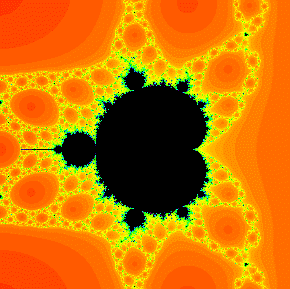##### A baby Mandelbrot set in the parameter space for z3 + C / z3. Note the red regions attached to the endpoints of certain antennas in this Mandelbrot set --- these are the "halos."
In this paper we prove the existence of "principal" Mandelbrot sets in the parameter planes for the functions zn + C / zn when n > 2. We also show that each of these Mandelbrot sets has infinitely many halos attached. These halos are Sierpinski holes in which the corresponding maps have Julia sets that are Sierpinski curves. The halos are attached at the endpoints of the antennas of the Mandelbrot set corresponding to Misiurewicz parameters where (in the quadratic polynomial case) the external rays of argument p/2k would land. In Complex Dynamics: Twenty-Five Years After the Appearance of the Mandelbrot Set, American Mathematical Society. Contemporary Math, 396, 2006, 37-50.

### Sierpinski Carpets and Gaskets As Julia Sets of Rational Maps

With Paul Blanchard, Daniel M. Look, Mónica Moreno Rocha, Stefan Siegmund, Pradipta Seal, and David Uminsky##### The parameter plane for the family of functions z2 + C / z2.
In this paper we give a survey of the dynamical and parameter planes for the family of rational maps

Fc(z) = z2 + c/z2.

In Dynamics on the Riemann Sphere, ed., P. Horth and C. Petersen. European Math Society, 2006, 97-119.

### A Criterion for Sierpinski Curve Julia Sets

With Daniel M. Look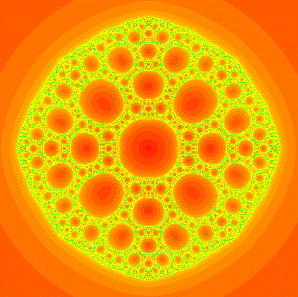##### A Sierpinski curve Julia set for the function z2 -1 / 16 z2.

In this paper we prove that, for the family of rational maps

Fc(z) = z2 + c/z2,
if the critical points have orbits that eventually tend to infinity (i.e., the critical points do not lie in the immediate basin of infinity), then the Julia set is a Sierpinski curve. We also show that, when the Julia set of Fc is connected, the complement of the closure of the basin of infinity consists of just one component. Topology Proceedings 30 (2006), 163-179.

### Structure of the McMullen Domain in the Parameter Planes for Rational Maps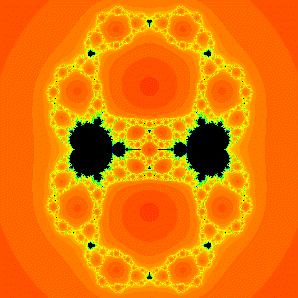##### The parameter space for the family of rational maps z3 + A / z3. The central hole in this image is the McMullen domain.
In this paper we prove that the McMullen domain for the family of rational maps zn + C / zn when n > 2 is a single simply connected open subset of the parameter plane. We also show that this domain is bounded by a simple closed curve. Here the McMullen domain consists of those parameter values for which the Julia set is a Cantor set of simple closed curves. Fundamenta Mathematicae. 185 (2005), 267-285.

### Indecomposable Continua and Misiurewicz Points in Exponential Dynamics

With Xavier Jarque and Mónica Moreno Rocha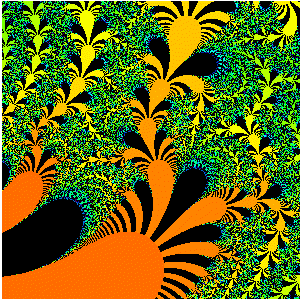##### A Julia set for a complex exponential function.
In this paper we describe several new types of invariant sets that appear in the Julia sets of the complex exponential function c ez. These sets include indecomposable continua formed by a pair of hairs that accumulate upon one another, and also another indecomposable continuum which is the set of accumulation points of a completely separate hair. International Journal of Bifurcation and Chaos 15 (2005), 3281-3293.

### Buried Sierpinski Curve Julia Sets

With Daniel M. Look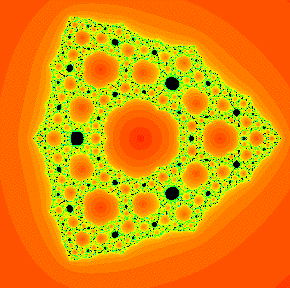##### A buried Sierpinski curve Julia set for the function z2 -0.328 / z.
In this paper we prove the existence of a different kind of Sierpinski curve Julia set from the ones described in earlier papers. These Sierpinski curves have complementary domains that consist not only of the basin of attraction of infinity, but also of the basins of other finite attracting cycles. Discrete and Continuous Dynamical Systems. 13 (2005), 1035-1046.

### Sierpinski Curve Julia Sets and Singular Perturbations of Complex Polynomials

With Paul Blanchard, Daniel M. Look, Pradipta Seal, and Yakov Shapiro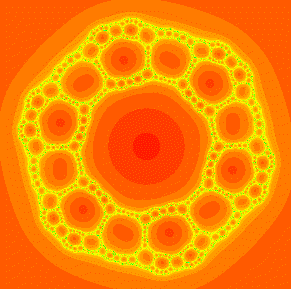##### Another example of a Sierpinski curve Julia set, this time from the family z3 + C / z3.
In this paper we describe a family of rational maps of the complex plane that feature infinitely many distinct parameter values for which the corresponding Julia set is a Sierpinski curve (sometimes called a Sierpinski carpet). Although all of these Julia sets are homeomorphic, we prove that there are infinitely many open sets in the parameter plane in which the corresponding maps on the Sierpinski curve have different (non-conjugate) dynamics. Ergodic Theory and Dynamical Systems 25 (2005), 1047-1055.

### The Escape Trichotomy for Singularly Perturbed Rational Maps

With Daniel M. Look and David Uminsky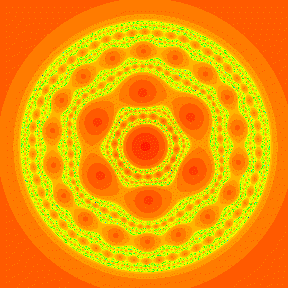##### An example of a Julia set that is a Cantor set of Jordan curves. The map here is z3 + (0.006 + 0.002 i) / z3.
In this paper we discuss a trichotomy that occurs in the topology of the Julia sets of rational maps of the form zn + A / zd. We show that, depending upon how the critical orbit escapes to infinity, the Julia set is either a Cantor set, a Sierpinski curve, or a Cantor set of circles. Indiana University Mathematics Journal 54 (2005), 1621-1634.

### Symbolic Dynamics for a Sierpinski Curve Julia Set

With Daniel M. Look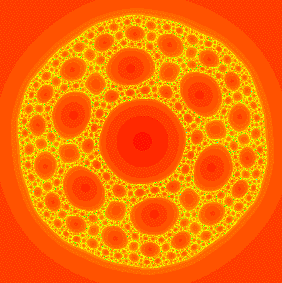##### Another example of a Sierpinski curve Julia set also drawn from the family z3 + C / z3.
In this paper we describe the dynamics of a particular member of the family of rational maps of the complex plane given by F(z) = z2 + c / z2 . For this family there are infinitely many distinct parameter values for which the corresponding Julia set is a Sierpinski curve (sometimes called a Sierpinski carpet). Using symbolic dynamics, we analyze completely the case where c = -1/16. This is the typical case in this family; the dynamics on all other Julia sets may be handled in similar fashion. Journal of Difference Equations and Applications. 11 (2005), 581-596.

### Cantor and Sierpinski, Julia and Fatou: Complex Topology Meets Complex Dynamics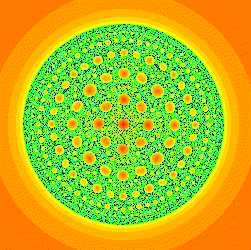##### Yet another Sierpinski curve Julia set drawn from the family of functions z2 + C / z2.

In this paper we describe several examples of interesting topological spaces (Sierpinski curves, Cantor bouquets, and indecomposable continua) that also arise as the Julia sets of complex functions. Notices of the American Mathematical Society. 51 (2004), 9-15.

Color Figures:

### Topological Bifurcations

This paper surveys some of the surprising changes in the topological structure of Julia sets for entire functions that occur when parameters are varied. Topology Proceedings. 28 (2004), 99-112.

### Chaos Rules!

This paper is a survey paper written for undergraduates describing the results of various chaos games. Math Horizons, November (2004), 11-14.

### Fractal Patterns and Chaos Games

This paper is a survey paper written for high school teachers and describing the results of various chaos games. Mathematics Teacher. 98 (2004), 228-233.

### Singular Perturbations of Quadratic Maps

With Kresimir Josic and Yakov Shapiro

In this paper we describe the global bifurcations in the family F(z) = z2 + c/z, i.e., the singular pertubation of z2 obtained by adding a pole at the origin. International Journal of Bifurcation and Chaos 14 (2004), 161-169.

### Playing Catchup with Iterated Exponentials

With Kresimir Josic, Mónica Moreno Rocha, Pradipta Seal, Yakov Shapiro, and Alex Frumosu

In this paper we discuss an interesting phenomenon in the exponential family where certain exponential functions have orbits that can "catch up" with other, supposedly faster orbits. The American Mathematical Monthly 111 (2004), 704-708.

### A Survey of Exponential Dynamics

In this paper we review several interesting dynamical and topological constructions dealing with the complex exponential function K ez where K > 0. In: New Progress in Difference Equations, ed. B. Aulbach, S. Elaydi, and G. Ladas. Chapman & Hall/CRC (2004), 105-122.

### Complex Dynamics and Symbolic Dynamics

With Paul Blanchard and Linda Keen

This is a summary of a lecture that was presented at Short Course on Symbolic Dynamics and Its Applications held at the joint AMS-MAA meeting in San Diego in January, 2002. In Symbolic Dynamics and its Applications, Proceedings of the Symposia in Applied Mathematics 60 (2004), 37-60.

### A Semilinear Model for Exponential Dynamics and Topology

With Mónica Moreno Rocha

We describe a family of semilinear maps of a strip in the plane, each of which features an invariant indecomposable continuum. The dynamics on and topology of these continua resemble those of the complex exponetial family Ek(z) = cez. In Topology Proceedings 26 (2002), 153-167.

### Indecomposable Continua in Exponential Dynamics

With Xavier Jarque

We show that there are many itineraries for the complex exponential map for which the corresponding set of points is an indecomposable continuum, not a hair, as occurs in the case of bounded regular sequences. The itineraries for which this occurs include bounded sequences which consist of longer and longer blocks of zeroes, the length of which grows quickly. Conformal Geometry and Dynamics 6 (2002), 1-12.

### Hyperbolic Components of the Complex Exponential Family

With Núria Fagella and Xavier Jarque

We describe the hyperbolic components of the parameter plane for the complex exponential family using two tools: a parameter plane kneading sequence and a dynamical plane kneading sequence. We prove the existence of a hyperbolic component with any parameter plane kneading sequence and investigate the relationship between these two sequences. In Fundamenta Mathematicae 174 (2002), 193-215.

### Geometry of the Antennas in the Mandelbrot Set

With Mónica Moreno Rocha

This paper proves that one can determine (using harmonic measure) the p/q bulb in the Mandelbrot set by looking at the geometry of its antenna. Fractals. 10 (2002), 39-46.

### Accessible Points in the Julia Sets of Stable Exponentials

With Ranjit Bhattacharjee, R. E. Lee Deville, Kresimir Josic, and Mónica Moreno Rocha

We discuss which points in the Julia set of a complex exponential map are accessible from the basin of attraction of an attracting cycle. Basically, only certain of the endpoints of hairs in this Cantor bouquet are accessible. Those endpoints that are accesssible are determined by the kneading sequence of the map. Discrete and Continuous Dynamical Systems. 1 (2001), 299-318.

### Homoclinic Points in Complex Dynamical Systems

We prove that a saddle-node (parabolic) periodic point in a complex dynamical system often admits homoclinic points, and in the case that these homoclinic points are nondegenerate, this is accompanied by the existence of infinitely many baby Mandelbrot sets converging to the saddle-node parameter value in the corresponding parameter plane. In Global Analysis of Dynamical Systems, ed.: H. Broer, B. Krauskopf, G. Vegter. IOP Publishing (2001), 329-338.

### Tying Hairs for Structurally Stable Exponentials

With Ranjit Bhattacharjee

In this paper we discuss the kneading sequence for complex exponential maps and show how this sequence can be used to determine how many hairs are tied together in the Julia sets of these maps. Ergodic Theory and Dynamical Systems 20 (2000), 1603-1617.

### Convergence of Polynomials to the Exponential

With Clara Bodelon, Lisa Goldberg, Michael Hayes, John Hubbard, and Gareth Roberts

This paper investigates how the parameter plane for the degree d polynomial family C(1 + x/d)d is related to the corresponding plane for the exponential family Cez. Journal of Difference Equations and Applications 6 (2000), 275-307.

### Hairs for the Complex Exponential Family

With Clara Bodelon, Lisa Goldberg, Michael Hayes, John Hubbard, and Gareth Roberts

In this paper we prove the existence of invariant curves or "hairs" that lie in the Julia set of the complex exponential family EK(z) = Kez. We also discuss similar curves that lie in the parameter plane for this family. On these curves, the Julia set of the corresponding exponential is the entire complex plane. International Journal of Bifurcation and Chaos. 9 (1999), 1517-1534.

### Cantor Bouquets, Explosions, and Knaster Continua: Dynamics of Complex Exponentials

This paper investigates several interesting aspects of the topology of the Julia set of the complex exponential family. Publicacions Matematiques. 43 (1999), 27-54.

### The Mandelbrot Set and the Farey Tree

This paper discusses and makes precise several of the "folk theorems" regarding the size of various portions of the Mandelbrot set. American Mathematical Monthly 106 (1999), 289-302.

Complete list of publications